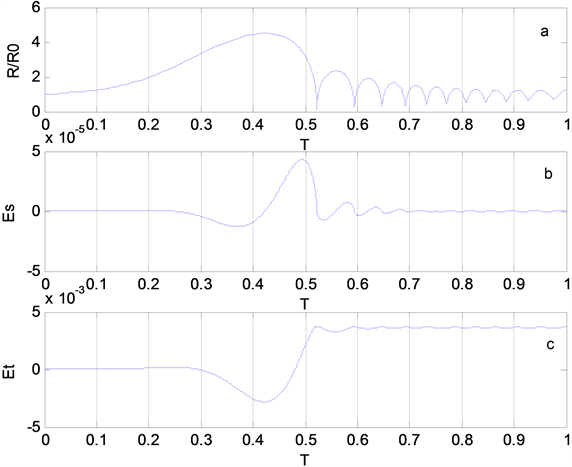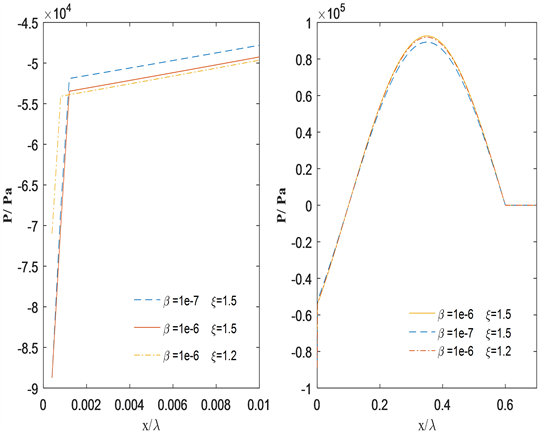# 含气泡液体中声场能量的传播Propagation of Sound Energy in Bubbly Liquids

• 全文下载: PDF(600KB)    PP.169-174   DOI: 10.12677/OJAV.2019.74019
• 下载量: 112  浏览量: 153   国家自然科学基金支持

Aiming at the problem of sound wave propagation in bubbly liquids, an idea of using energy propagation to establish a model is proposed. The vibration of the bubble and the mechanical energy of the sound are combined to establish an energy propagation model of the sound field in the bubbly liquids, and the model is used as a single numerical simulation of the mechanical energy change of the bubble caused by bubble cavitation, the mechanical energy loss of the driven sound field and the influence of bubble vibration on the propagation process of the sound field during the model Through numerical analysis, it’s found that the more the volume change of a single bubble during the vibration process, the more obvious the change of mechanical energy, and the greater the mechanical energy loss of the corresponding driving sound field; if the bubble is cavitation under the action of the driving sound field, a small cavitation area is formed near the sound source, and the size of the area is affected by the number of bubbles and the intensity of the sound field. Finally, the proposed and applied sound field energy propagation model in bubbly liquids can be conveniently used to deal with the propagation of sound fields in bubbly liquids.

1. 引言

2. 声场能量传播模型

2.1. 气泡振动的能量模型

$\rho \left(R\stackrel{¨}{R}+\frac{3}{2}{\stackrel{˙}{R}}^{2}\right)={P}_{g}-\frac{2\sigma }{R}-4\mu \frac{\stackrel{˙}{R}}{R}-P$ (1)

$P={P}_{\infty }+{P}_{r}$ (2)

${P}_{g}=\left({P}_{0}+\frac{2\delta }{{R}_{0}}\right){\left(\frac{{R}_{0}}{R}\right)}^{3\gamma }$ (3)

$P\cdot dV=-\rho \left(R\stackrel{¨}{R}+\frac{3}{2}{\stackrel{˙}{R}}^{2}\right)\cdot dV+{P}_{g}\cdot dV-\frac{2\delta }{R}\cdot dV-\frac{4\mu \stackrel{˙}{R}}{R}\cdot dV-{P}_{\infty }\cdot dV$ (4)

2.2. 声场能量传播模型

${E}_{0}=\frac{{V}_{0}}{2}\rho \left({\upsilon }^{2}+\frac{1}{{\rho }^{2}{c}^{2}}{P}_{r}^{2}\right)$ (5)

$\Delta E=\frac{3\beta {V}_{0}}{4\pi {R}_{0}^{3}}{P}_{r}\cdot dV$ (6)

${E}^{\prime }={E}_{0}-\Delta E$ (7)

$\frac{{V}_{0}}{2}\rho \left({\upsilon }^{2}+\frac{1}{{\rho }^{2}{c}^{2}}{P}_{r}{}^{2}\right)-\frac{3\beta {V}_{0}}{4\pi {R}_{0}^{3}}{P}_{r}\cdot dV=\frac{{V}_{0}}{2}\rho \left({{\upsilon }^{\prime }}^{2}+\frac{1}{{\rho }^{2}{c}^{2}}{{P}^{\prime }}_{r}^{2}\right)$ (8)

3. 数值模拟分析Figure 1. Bubble radius, mechanical energy and mechanical energy loss of driving sound field change with timeFigure 2. Influence of bubble content and sound field amplitude on sound field propagation

4. 结论

  Jamshidi, R. and Brenner, G. (2013) Dissipation of Ultrasonic Wave Propagation in Bubbly Liquids Considering the Ef-fect of Compressibility to the First Order of Acoustical Mach Number. Ultrasonics, 53, 842-848. https://doi.org/10.1016/j.ultras.2012.12.004  王勇, 林书玉, 张小丽. 声波在含气泡液体中的线性传播[J]. 物理学报, 2013, 62(6): 064304.  王勇, 林书玉, 张小丽. 含气泡液体中的非线性声传播[J]. 物理学报, 2014, 63(3): 4034301.  Ashokkumar, M. (2011) The Characterization of Acoustic Cavitation Bubbles—An Overview. Ultrasonics Sonochemistry, 18, 864-872. https://doi.org/10.1016/j.ultsonch.2010.11.016  沈壮志, 吴胜举. 声场与电场作用下空化泡的动力学特性[J]. 物理学报, 2012, 61(12): 352-357.  王成会, 莫润阳, 胡静, 等. 泡群内气泡振动特性分析[J]. 中国科学: 物理学力学天文学, 2017, 47(2): 59-67.  马艳. 声场中空化气泡的耦合振动及形状不稳定性的研究[J]. 物理学报, 2018, 67(3): 124-132.  Vanhille, C. and Campos-Pozuelo, C. (2012) Acoustic Cavitation Mechanism: A Nonlinear Model. Ultrasonics Sonochemistry, 19, 217-220. https://doi.org/10.1016/j.ultsonch.2011.06.019  Louisnard, O. (2012) A Simple Model of Ultrasound Propaga-tion in a Cavitating Liquid. Part I: Theory, Nonlinear Attenuation and Traveling Wave Generation. Ultrasonics Sono-chemistry, 19, 56-65. https://doi.org/10.1016/j.ultsonch.2011.06.007  Vanhille, C. and Campos-Pozuelo, C. (2013) Numerical Simu-lations of Three-Dimensional Nonlinear Acoustic Waves in Bubbly Liquids. Ultrasonics Sonochemistry, 20, 963-969. https://doi.org/10.1016/j.ultsonch.2012.11.009  Gumerov, N., Ohl, C.D., Akhatov, I.S., et al. (2013) Waves of Acoustically Induced Transparency in Bubbly Liquids: Theory and Experiment. Proceedings of Meetings on Acoustics, 19, Article ID: 045012. https://doi.org/10.1121/1.4799398  Yasui, K., Towata, A., Tuziuti, T., et al. (2011) Effect of Static Pressure on Acoustic Energy Radiated by Cavitation Bubbles in Viscous Liquids under Ultrasound. The Journal of the Acoustical Society of America, 130, 3233-3242. https://doi.org/10.1121/1.3626130  Prosperetti, A. and Lezzi, A. (1986) Bubble Dynamics in a Compressible Liquid. Part 1. First-Order Theory. Journal of Fluid Mechanics, 168, 457-478. https://doi.org/10.1017/S0022112086000460  Foldy, L.L. (1945) The Multiple Scattering of Waves. I. General Theory of Isotropic Scattering by Randomly Distributed Scatterers. Physical Review, 67, 107-119. https://doi.org/10.1103/PhysRev.67.107  Leighton, T.G. (1997) The Acoustic Bubble. Academic Press, Lon-don.  吴文华, 翟薇, 胡海豹, 等. 液体材料超声处理过程中声场和流场的分布规律研究[J]. 物理学报, 2017, 66(19): 148-156.